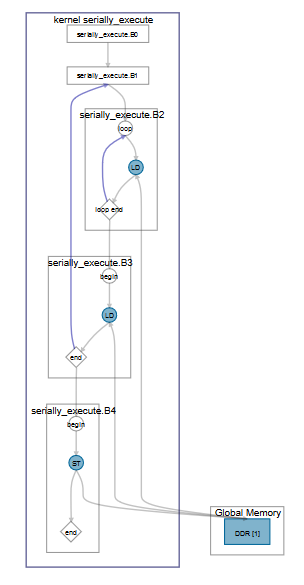ID 683521
Date 12/19/2022
Public

## 3.4.3. Nested Loops

The Intel® FPGA SDK for OpenCL™ Offline Compiler does not infer pipelined execution because of the ordering of loop iterations. As a result, outer loop iterations might be out of order with respect to the ensuing inner loops because the number of iterations of the inner loops might differ for different out loop iterations.

To solve the problem of out-of-order outer loop iterations, design inner loops with lower and upper bounds that do not change between outer loop iterations.

### Single Work-Item Execution

To ensure high-throughput single work-item-based kernel execution on the FPGA, the Intel® FPGA SDK for OpenCL™ Offline Compiler must process multiple pipeline stages in parallel at any given time. This parallelism is realized by pipelining the iterations of loops.

Consider the following simple example code that shows accumulating with a single-work item:

1 kernel void accum_swg (global int* a,
global int* c,
int size,
int k_size) {
2     int sum;
3     for (int k = 0; k < k_size; ++k) {
4        for (int i = 0; i < size; ++i) {
5            int j = k * size + i;
6            sum[k] += a[j];
7        }
8     }
9     for (int k = 0; k < k_size; ++k) {
10       c[k] = sum[k];
11    }
12 }
During each loop iteration, data values from the global memory a is accumulated to sum[k]. In this example, the inner loop on line 4 has an initiation interval value of 1 with a latency of 11. The outer loop also has an initiation interval value greater than or equal to 1 with a latency of 8.
Note: The launch frequency of a new loop iteration is called the initiation interval (II). II refers to the number of hardware clock cycles for which the pipeline must wait before it can process the next loop iteration. An optimally unrolled loop has an II value of 1 because one loop iteration is processed every clock cycle.
Figure 60. System View of Single-Work Item KernelThe following figure illustrates how each iteration of i enters into the block:

Figure 61. Inner Loop accum_swg.B2 Execution

When you observe the outer loop, having an II value of 1 also means that each iteration of the thread can enter at every clock cycle. In the example, k_size of 20 and size of 4 is considered. This is true for the first eight clock cycles as outer loop iterations 0 to 7 can enter without any downstream stalling it. Once thread 0 enters into the inner loop, it takes four iterations to finish. Threads 1 to 8 cannot enter into the inner loop and they are stalled for four cycles by thread 0. Thread 1 enters into the inner loop after thread 0's iterations are completed. As a result, thread 9 enters into the outer loop on clock cycle 13. Threads 9 to 20 enters into the loop at every four clock cycles, which is the value of size. Through this example, you can observe that the dynamic initiation interval of the outer loop is greater than the statically predicted initiation interval of 1 and it is a function of the trip count of the inner loop.

Figure 62. Single Work-Item Execution### Nonlinear Execution

Loop structure does not support linear execution. The following code example shows that the outer loop i contains two divergent inner loops. Each iteration of the outer loop may execute one inner loop or the other, which is a nonlinear execution pattern.

__kernel void structure (__global unsigned* restrict output1,
__global unsigned* restrict output2,
int N) {
for (unsigned i = 0; i < N; i++) {
if ((i & 3) == 0) {
for (unsigned j = 0; j < N; j++) {
output1[i+j] = i * j;
}
}
else
{
for (unsigned j = 0; j < N; j++) {
output2[i+j] = i * j;
}
}
}
}

### Serial Regions

Serial region might occur in nested loops when an inner loop access causes an outer loop dependency. The inner loop becomes a serial region in the outer loop iteration due to data or memory dependencies.

At steady state, the II of outer loop = II of inner loop * trip count of inner loop. For inner loops with II greater than 1 and outer loop that has no serially executed regions, it is possible to interleave threads from the outer loop.

Consider the following code example:

kernel void serially_execute (global int * restrict A,
global int * restrict B,
global int * restrict result,
unsigned N) {
int sum = 0;
for (unsigned i = 0; i < N; i++) {
int res;
for (int j = 0; j < N; j++) {
sum += A[i*N+j];
}
sum += B[i];
}
*result = sum;
}
In the example, the dependence in the outer loop resulted in the serial execution of the inner loop. The main difference in performance is the steady state II of outer loop = II of inner loop * (trip count of inner loop - 1) + latency. In this example, II of inner loop is 1 with latency of 4 and II of outer loop is 1 with latency of 7. If N is large, such as 400, when compared to latency, then serial execution has little impact from the outer loop II.
Figure 63. System View of the KernelFigure 64. Serial Execution